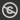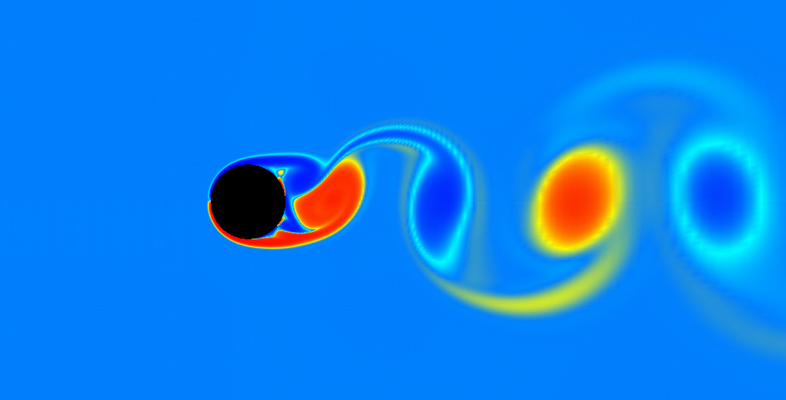## Free courses

Produced by The Open University, a world leader in open and distance learning, all OpenLearn courses are free to study. We offer nearly 1000 free courses across 9 different subject areas. Our courses are available to start right away.

• All topics
Results: 215 itemsfree course icon level 1: introductory icon

Science, Maths & Technology

### Scales in space and time

Scale – dealing with the miniscule to the massive, over milliseconds to millennia – is central to all the sciences. In this free course, Scales in space and time, you’ll go to the absolute limits of what can be measured – all through a study of an oak tree! By studying it you will be introduced to concepts in physics, chemistry, biology and ...

Free course
12 hrsfree course icon level 3: advanced icon

Science, Maths & Technology

### Exploring the relationship between anxiety and depression

This free advanced level course, Exploring the relationship between anxiety and depression, serves as an introduction to masters level study in neurosciences and mental health. Focusing on anxiety and depression, you will consider key issues concerning diagnosis, causes and interventions, exploring how these conditions relate to each other. You ...

Free course
3 hrsfree course icon level 2: intermediate icon

Science, Maths & Technology

### Kinematics of fluids

This free course examines the basic kinematics of two-dimensional fluid flows. Section 1 introduces the diﬀerential equations for pathlines and streamlines. Section 2 introduces a scalar ﬁeld, called the stream function, which for an incompressible ﬂuid provides an alternative method of modelling the ﬂow and ﬁnding the streamlines. Sections 2 ...

Free course
16 hrsfree course icon level 2: intermediate icon

Science, Maths & Technology

### Number theory

This free course is an introduction to Number Theory. Section 1 provides a brief introduction to the kinds of problem that arise in Number Theory. Section 2 reviews and provides a more formal approach to a powerful method of proof, mathematical induction. Section 3 introduces and makes precise the key notion of divisibility. The Division ...

Free course
16 hrsfree course icon level 2: intermediate icon

Science, Maths & Technology

### Group theory

This free course consolidates and builds on group theory studied at OU level 2 or equivalent. Section 1 describes how to construct a group called the direct product of two given groups, and then describes certain conditions under which a group can be regarded as the direct product of its subgroups. Section 2 describes the key properties of the ...

Free course
16 hrsfree course icon level 2: intermediate icon

Science, Maths & Technology

### Rings and polynomials

This free course contains an introduction to rings and polynomials. We see that polynomial rings have many properties in common with the integers; for example, we can define a division algorithm, and this enables us to develop the analogue of the highest common factor for two polynomials. Section 1 explores the abstract definitions of a ring ...

Free course
16 hrsfree course icon level 2: intermediate icon

Science, Maths & Technology

### Metric spaces and continuity

This free course contains an introduction to metric spaces and continuity. The key idea is to use three particular properties of the Euclidean distance as the basis for defining what is meant by a general distance function, a metric. Section 1 introduces the idea of a metric space and shows how this concept allows us to generalise the notion of ...

Free course
16 hrsfree course icon level 2: intermediate icon

Science, Maths & Technology

### Introduction to the calculus of variations

This free course concerns the calculus of variations. Section 1 introduces some key ingredients by solving a seemingly simple problem – finding the shortest distance between two points in a plane. The section also introduces the notions of a functional and of a stationary path. Section 2 describes basic problems that can be formulated in terms ...

Free course
16 hrsfree course icon level 2: intermediate icon

Science, Maths & Technology

### Linear programming – the basic ideas

This free course examines the formulation and solution of small linear programming problems. Section 1 deals with the formulation of linear programming models, describing how mathematical models of suitable real-world problems can be constructed. Section 2 looks at graphical representations of two-dimensional models, considers some theoretical ...

Free course
16 hrsfree course icon level 2: intermediate icon

Science, Maths & Technology

### Univariate continuous distribution theory

This free course looks at a number of the basic properties of statistical models. Section 1 is concerned with the distributions of continuous random variables which are described by their probability density functions (pdfs) and cumulative distribution functions (cdfs). Section 2 is concerned with moments and covers, the concept of expectation ...

Free course
10 hrsfree course icon level 2: intermediate icon

Science, Maths & Technology

### Point estimation

This free course looks at point estimation, that is, the estimation of the value of the parameter of a statistical model by a single number, a point estimate for the parameter. Section 1 develops some aspects of maximum likelihood estimation. In particular, you will find out how to obtain the maximum likelihood estimator of an unknown parameter,...

Free course
10 hrsfree course icon level 2: intermediate icon

Science, Maths & Technology

### Modelling events in time

This free course develops ideas about probability and random processes. Sections 1 and 2 introduce the fundamental ideas of random processes through a series of examples. Section 3 describes a model that is appropriate for events occurring ‘at random’ in such a way that their rate of occurrence remains constant. Section 4 derives the main ...

Free course
16 hrs